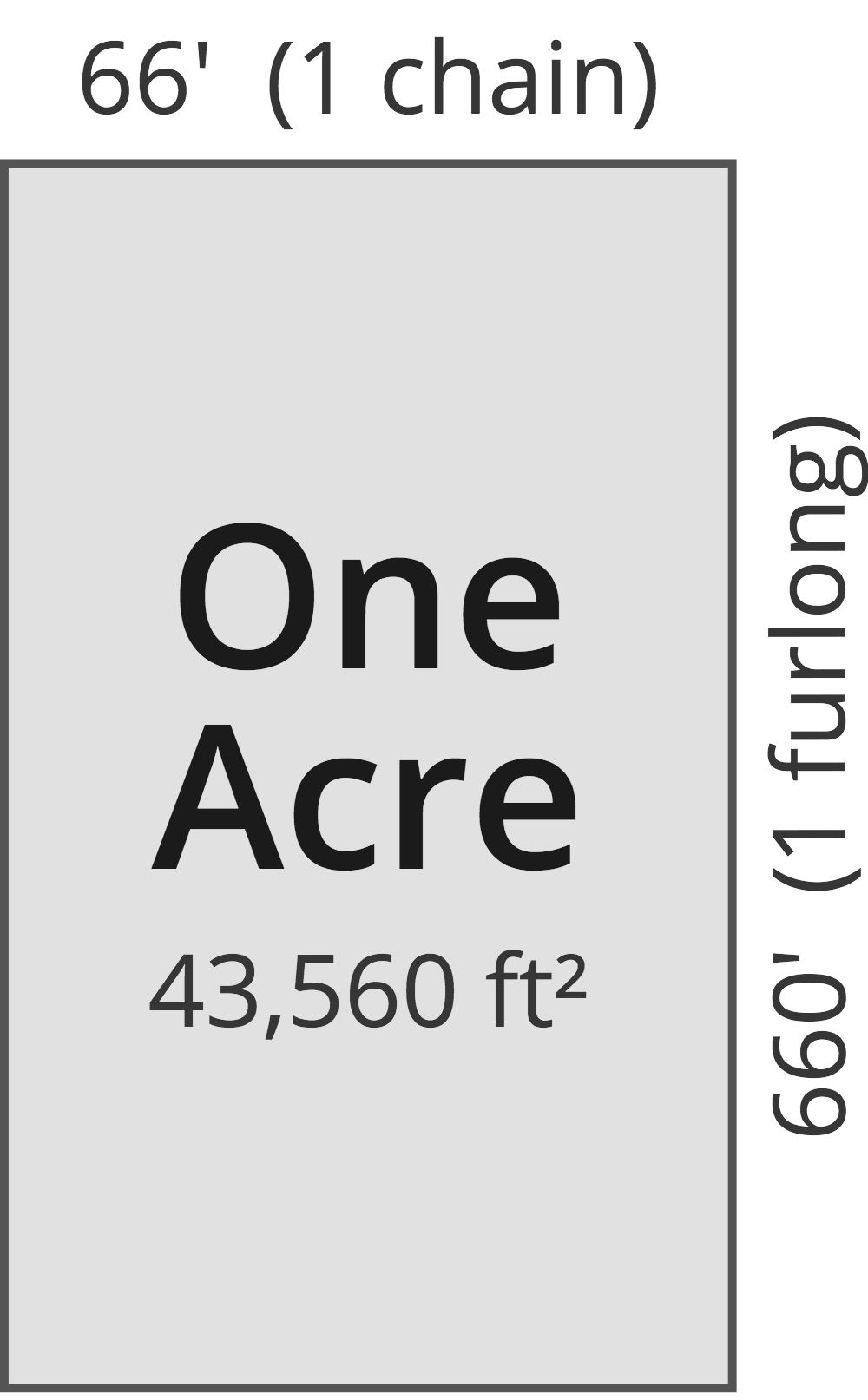# Square Meters to Acres Converter

Enter the area in square meters below to get the value converted to acres.

(find square meters)
Results in Acres:1 sq m = 0.000247 ac

Do you want to convert acres to square meters?

## How to Convert Square Meters to Acres

To convert a measurement in square meters to a measurement in acres, multiply the area by the following conversion ratio: 0.000247 acres/square meter.

Since one square meter is equal to 0.000247 acres, you can use this simple formula to convert:

acres = square meters × 0.000247

The area in acres is equal to the area in square meters multiplied by 0.000247.

For example, here's how to convert 5,000 square meters to acres using the formula above.
acres = (5,000 sq m × 0.000247) = 1.235527 ac

## What is a Square Meter?

One square meter is equivalent to the area of a square with sides that are each 1 meter in length. One square meter is equal to 10.76391 square feet or 10,000 square centimeters.

The square meter, or square metre, is the SI derived unit for area in the metric system. A square meter is sometimes also referred to as a square m. Square meters can be abbreviated as sq m, and are also sometimes abbreviated as . For example, 1 square meter can be written as 1 sq m or 1 m².

You can use a square meters calculator to calculate the area of a space if you know its dimensions.

## What is an Acre?

One acre is defined as the area equal to a space that is one chain (66 ft) by one furlong (660 ft), or 10 square chains. That's equal to 43,560 square feet or 1/640 of a square mile for those unfamiliar with those units of measure.The acre is a US customary and imperial unit of area. Acres can be abbreviated as ac; for example, 1 acre can be written as 1 ac.

You can use an acreage calculator to measure the area of a plot of land in acres by locating the boundaries on a map.

## Square Meter to Acre Conversion Table

Table showing various square meter measurements converted to acres.
Square Meters Acres
1 sq m 0.000247 ac
2 sq m 0.000494 ac
3 sq m 0.000741 ac
4 sq m 0.000988 ac
5 sq m 0.001236 ac
6 sq m 0.001483 ac
7 sq m 0.00173 ac
8 sq m 0.001977 ac
9 sq m 0.002224 ac
10 sq m 0.002471 ac
20 sq m 0.004942 ac
30 sq m 0.007413 ac
40 sq m 0.009884 ac
50 sq m 0.012355 ac
60 sq m 0.014826 ac
70 sq m 0.017297 ac
80 sq m 0.019768 ac
90 sq m 0.022239 ac
100 sq m 0.024711 ac
200 sq m 0.049421 ac
300 sq m 0.074132 ac
400 sq m 0.098842 ac
500 sq m 0.123553 ac
600 sq m 0.148263 ac
700 sq m 0.172974 ac
800 sq m 0.197684 ac
900 sq m 0.222395 ac
1,000 sq m 0.247105 ac
2,000 sq m 0.494211 ac
3,000 sq m 0.741316 ac
4,000 sq m 0.988422 ac
5,000 sq m 1.2355 ac
6,000 sq m 1.4826 ac
7,000 sq m 1.7297 ac
8,000 sq m 1.9768 ac
9,000 sq m 2.2239 ac
10,000 sq m 2.4711 ac

## References

1. Collins Dictionary, Definition of 'square meter', https://www.collinsdictionary.com/us/dictionary/english/square-meter
2. National Institute of Standards and Technology, Specifications, Tolerances, and Other Technical Requirements for Weighing and Measuring Devices, Handbook 44 - 2019 Edition, https://nvlpubs.nist.gov/nistpubs/hb/2019/NIST.HB.44-2019.pdf